## BINOMIALEDGE

 Sharpens (or blurs) an image near edges using a Binomial shaped filter.

USAGE: binomialedge [-w width] [-k kind] [-m mix] [-e edge] [-t threshold] [-b blur] infile outfile
USAGE: binomialedge [-h or -help]

-w ...... width ........... width of square filter; width=3, 5 or 7;
........................... default=3
-k ...... kind ............ kind=high or low (pass filter);
........................... default=high
-m ...... mix ............. mixing percent with original image;
........................... mix=integer 0 to 100; default=20
-e ...... edge ............ edge method used to threshold edges;
........................... edge=0 is grayscale thresholding;
........................... edge=1 is binary thresholding;
........................... edge=2 is no edge masking
........................... default=0
-t ...... threshold ....... percent edge threshold value; 0 to 100;
........................... default=25
-b ...... blur ............ edge blurring distance; float >= 0;
........................... default=1
-h ........................ get help information
-help ..................... get help information

PURPOSE: To sharpen (or blur) an image near edges using a Binomial shaped filter.

DESCRIPTION: BINOMIALEDGE generates an output image which sharpenes (or blurs) only near edges using a Binomial shaped filter. The script first generates a mix of the image with either a high pass sharpened version of the image or low pass blurred version of the image. A high pass edge image is then thresholded and used as a mask to blend the sharpened or blurred image with the original image.

The basic blended low pass filtering formula is F = (1-m)*I + m*L, where I is the original image, L is the Gaussian low pass filtered image and m = mix/100. When m=0, we get only the original image and when m=1, we get only the low pass Gaussian filtered image. For intermediate value of m, we get a blend of the image and the Gaussian low pass filtered image. For high pass filtering, we form the high pass filter by subtracting the low pass filter from the original Thus in the formula above L is replaced by H=(I-L), and as the high pass image is primarily black we simply add the percentage of the high pass image to the original image so that F = I + m*H, which also can be expressed as F = (1+m)*I - m*L. Once the high pass sharpened image or low pass blurred image is created, a high pass edge image is then thresholded and used as a mask to blend the sharpened or blurred image with the original image.

For width=3, the 1D binomial series is 1 2 1. For width=5, the 1D binomial series is 1 4 6 4 1. For width=7, the 1D binomial series is 1 6 15 20 15 6 1. To form the 2D binomial filters, the outer product of the 1D column with the 1D row is computed.

For width=3, this becomes:
1 2 1
2 4 2
1 2 1

For width=5, this becomes:

 1 4 6 4 1 4 16 24 16 4 6 24 36 24 6 4 16 24 16 4 1 4 6 4 1

For width=7, this becomes:

 1 6 15 20 15 6 1 6 36 90 120 90 36 6 15 90 225 300 225 90 15 20 120 300 400 300 120 20 15 90 225 300 225 90 15 6 36 90 120 90 36 6 1 6 15 20 15 6 1

ARGUMENTS:

-w width is dimension of a square convolution kernel. Width can be 3, 5 or 7. the default is 3. For example a width of 3 will generate a 3x3 convolution kernel.

-k kind ... KIND is the kind of filter, either a high pass or low pass filter are allowed. Thus kind=high or low. The default=high. A low pass filter will cause blurring and a high pass filtered image will produce sharpening.

-m mix ... MIX is the percentage mixing factor to use to blend the filtered result with the original image. For kind=low, a value of mix=0, results in the original image and a value of mix=100 results in a pure low pass filtered image. For low pass filtering, a larger value for mix will produce more blurring. For kind=high, the mix percentage of the high pass filtered image will be added to the original image. A value of mix=0, results in the original image. A larger value for mix will sharpen or highlight the edges more. The default is mix=20.

-e edge ... EDGE is the method used to threshold the standard deviation image to get an edge mask image for use in compositing the original and sharpened image. Vaules may be 0, 1 or 2. A value of 0 indicates that thresholding will be done using -black-threshold so that any value below the threshold is black but values above remain grayscale edges. A value of 1 indicates that thresholding will be done using -threshold to generate a binary edge mask image. A value of 2 indicates that no mask will be used. Thus sharpening (or blurring) will be done throughout the image. Value 0 produces the least edge sharpening (or blurring). Value 1 produces a greater amount of edge sharpening (or blurring). Value 2 sharpens (or blurs) throughout the image. The default is 0.

-t threshold ... THRESHOLD is the percentage threshold value to use. Values are integers between 0 and 100. The lower the threshold the more edges will be sharpened. Threshold is not needed or ignored for method=2. The default is 25.

-b blur ... BLUR is the edge blurring distance to spread the edges wider by a small amount. Values are floats >= 0. The default is 1.

CAVEAT: No guarantee that this script will work on all platforms, nor that trapping of inconsistent parameters is complete and foolproof. Use At Your Own Risk.

### EXAMPLES

 Binomial Edge Sharpening - Variation In Edge Masking Original ImageArguments: -e 0 Arguments: -e 1 Arguments: -e 2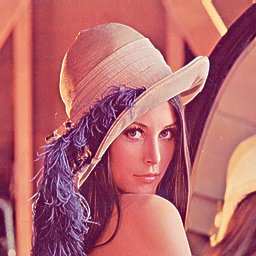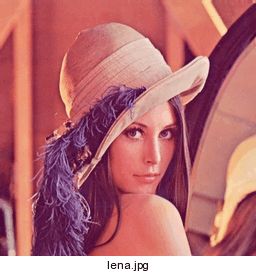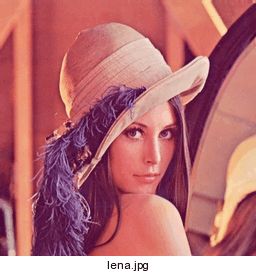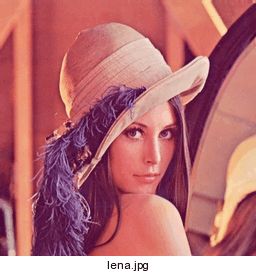Binomial Edge Sharpening - Variation In Mix Factor Original ImageArguments: -m 10 Arguments: -m 20 Arguments: -m 30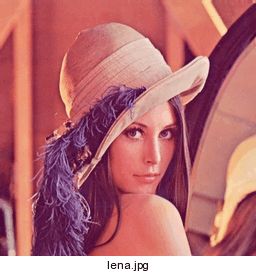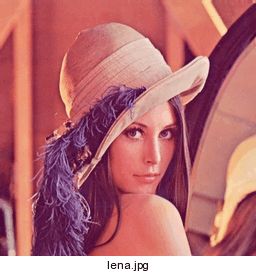What the script does is as follows for a edge sharpened image: Computes a thresholded edge image. Computes a high pass filtered non-thresholded edge image Blends the original with the non-thresholded edge image to make the sharpened image Blends the original with sharpened image using the thresholded edge image as a mask This is equivalent to the following IM commands for the case of binomial edge sharpening (-w 3 -m 20 -e 0 -t 25 -b 1): bin3="1,2,1,2,4,2,1,2,1" convert \$infile \( \$infile -convolve "\$bin3" \) -compose minus +swap -composite \ -colorspace Gray -blur 0x1 -contrast-stretch 0% -black-threshold 25% \$tmp0 convert \$infile \( \$infile -convolve "\$bin3" \) -compose minus +swap -composite \ -normalize \$tmp1 composite -blend 20%x100% \$tmp1 \$infile -matte \$tmp1 convert \$infile \$tmp1 \$tmp0 -composite \$outfile